# Sentence Case in ExcelArticle byExcelMojo Team## What Is Sentence Case In Excel?

The sentence case in Excel is a feature that enables one to change the case of a given text. And Excel offers three inbuilt functions, UPPER, LOWER, and PROPER, as part of the sentence case feature.

Users can use the sentence case change functions to update and view the given text in uppercase, lowercase, proper case, or a combination of different cases.

For example, the table below contains text data.

The requirement is to change the case of the given text as per the descriptions in column B and display the result in column C.

Then, we can apply sentence case in Excel cell range C2:C4 and obtain the required output.

While the UPPER() and LOWER() change the existing text case to uppercase and lowercase, the PROPER() displays the first letter of each word in the text in uppercase.

###### Key Takeaways
• The feature sentence case in Excel includes inbuilt functions, LOWER, UPPER, and PROPER, which help update the case of the given text.
• Users can apply the abovementioned functions to view the given text in uppercase, lowercase, title case, or a combination of the three cases.
• The LOWER, UPPER and PROPER Excel functions accept one argument, text, as input. And the argument value can be the text or a cell reference to the text.
• To draw practical solutions, you can apply the sentence case change functions with other inbuilt functions, such as SUBSTITUTE, LEFT, and LEN.

### Sentence Case() Excel Formula

The formulas for Excel sentence case are as follows:

1. LOWER()

The LOWER excel function is:

where,

• text: The text value or excel cell reference to the text value for which we require to update the case to lowercase.
1. UPPER()

The UPPER excel function is:

where,

• text: The text value or cell reference to the text value for which we require to update the case to uppercase.
1. PROPER()

The PROPER excel function is:

where,

• text: The text value or cell reference to the text value for which we require to update the case to title case.

The argument in each sentence case change function is mandatory.

Further, when applying the above functions in a cell, we can supply a single cell value as the argument. But when applying the functions as array formulas in excel, the functions accept a cell range of text values.

Also, unlike MS Word, there is no Excel sentence case shortcut. But we can create keyboard shortcuts or shortcuts in the Quick Access Toolbar using Excel VBA macros. But If we create a keyboard shortcut and the shortcut already exists, it will override the old one.

### How To Change Sentence Case In Excel?

We can apply sentence case in Excel to change a text case in two ways, namely:

1. Access from the Excel ribbon.
2. Enter in the worksheet manually.

We shall see the two methods for LOWER().

#### Method #1 – Access From The Excel Ribbon

Choose the target cell for output → select the Formulas tab → click the Text option drop-down → select the LOWER function, as shown below.

The Function Arguments window opens up. Enter the argument in the Text field → click OK, as depicted below.

#### Method #2 – Enter In The Worksheet Manually

1. Choose the target cell for the output.
2. Type =LOWER( in the cell. [Alternatively, type =L or =LO and double-click the LOWER function from the Excel suggestions.]
3. Enter the argument as a cell value or reference and close the brackets.
4. Press Enter to execute and view the given text in lowercase.

Likewise, we can follow the above methods to use the UPPER and PROPER functions in Excel. But the only difference is that we will have to navigate the path FormulasText and select the required function in place of the LOWER().

The below image shows three tables.

While the first and second tables contain a measurement unit and an acronym, the third table shows an employee name.

Suppose the requirement is to show the measurement unit in lowercase, the acronym in uppercase, and the employee’s name in title case. And the target cells are C3, C6, and C9.

Steps to change the sentence case are as follows –

1. Select the target cell C3 and enter the LOWER().

=LOWER(B3)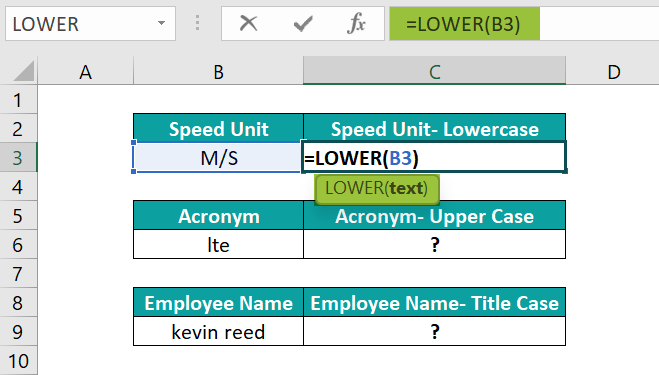Entering the below formula will give the same result.
=LOWER(“M/S”)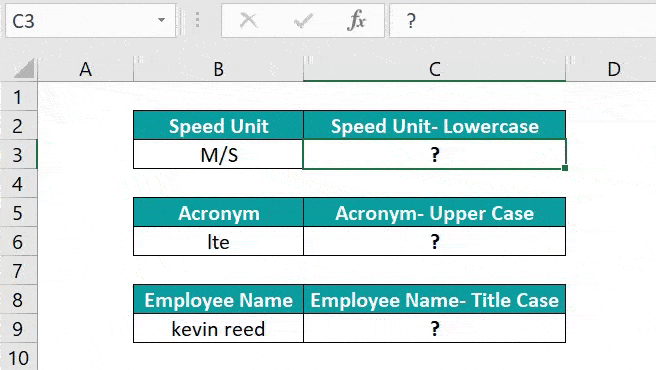2. Press Enter to view the text in lowercase.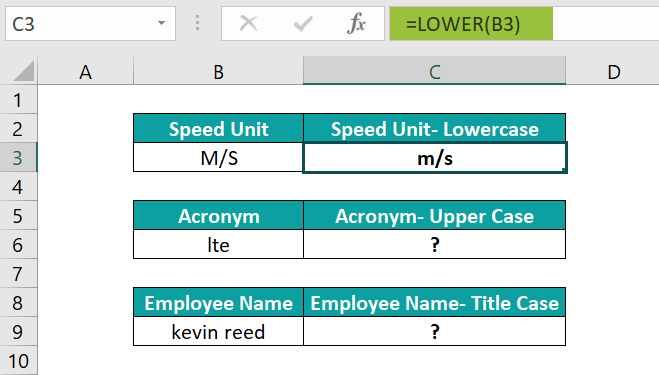Alternatively, we can select the target cell C3, and navigate the path FormulasTextLOWER to apply the LOWER function in the chosen cell.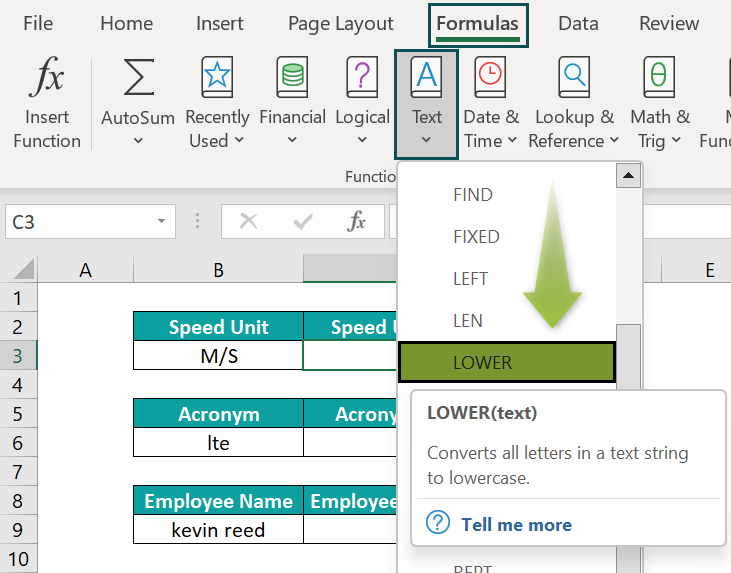The above step will open the Function Arguments window.

Next, enter the given cell reference in the respective field in the Function Arguments window.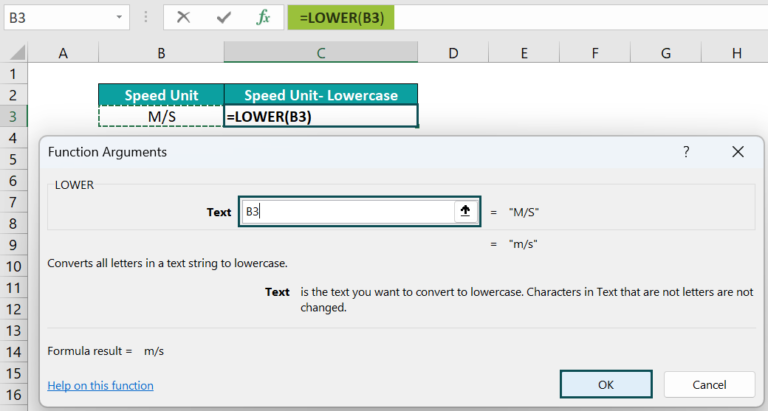Finally, click OK. The window will close, and we will obtain the LOWER() output in the target cell C3.]

So, the function accepts the cell reference to the given text as input and returns the text in lowercase.

3. Select the target cell C6, enter the UPPER(), and press Enter.

=UPPER(B6)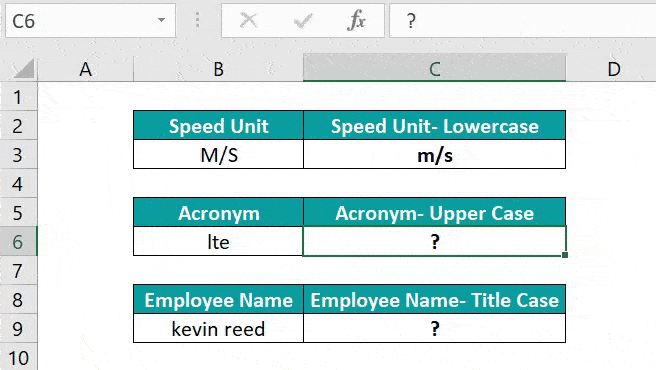The UPPER() changes the given acronym case into uppercase.

4. Select the target cell C9, enter the PROPER(), and press Enter.

=PROPER(B9)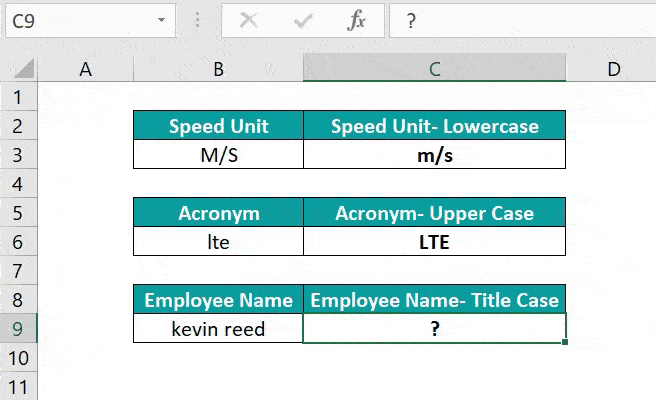The PROPER() shows the input text in the title case, with the first letter of each word in the text being in uppercase and the remaining characters in lowercase.

### Examples

The following Excel sentence case examples will help us utilize them in the best possible ways.

#### Example #1

The following example explains how to change the text in all caps to sentence case in Excel, with only the first character in uppercase.

The below table contains a list of quotes in uppercase.

Suppose the requirement is to show the text values in column C, with only the first letter in each text in uppercase.

Then, the steps to achieve the required output are as follows:

• Step 1: Select the target cell C3, enter the below formula, and press Enter.

=LEFT(B3,1)&LOWER(RIGHT(B3,LEN(B3)-1))

Let us consider the cell C7 expression to understand how the formula works.

First, the LEFT excel function returns the first character in the given text, “W”. Next, the LEN() returns the length of the given text, 44. So, the second argument in the RIGHT excel function becomes 43. And hence, the RIGHT() accepts the cell B7 text and returns 43 characters from the right end, leaving out the first character in the text, “W”.

Finally, the formula concatenates the two functions, LEFT, and RIGHT, output. And the result is a text with its case changed from all caps to sentence case in Excel, having only the first letter in uppercase.

#### Example #2

We can use the functions for updating the Excel sentence casewhen the supplied text is a paragraph containing more than one sentence.

For example, the below table contains a paragraph with all the sentences in lowercase.

Suppose we need to show the input text in cell C3, with each sentence in the paragraph starting with a capital letter.

Then, we can use PROPER() with SUBSTITUTE excel function in the target cell to achieve the desired outcome.

• Step 1: Select the target cell C3, enter the below formula, and press Enter.

=SUBSTITUTE(SUBSTITUTE(PROPER(SUBSTITUTE(SUBSTITUTE(SUBSTITUTE(B3,”. “,”1×1″),” “,”xjx”),”1×1″,”. “)),”xjx”,” “),”Xjx”,” “)

The inner-most SUBSTITUTE() replaces “. “ in the text with “1×1”. Next, the immediate outer SUBSTITUTE() substitutes “ “ in the resulting text with “xjx”. And the next outer SUBSTITUTE() replaces “1×1” in the text obtained in the previous step by “. “.

The above steps ensure that each sentence in the paragraph appears as a continuous text without spaces. And there is only a full stop and a space separating the sentences. So, the PROPER() changes the case of the first letter of the first sentence and the character after each full stop to uppercase.

Finally, the two outer SUBSTITUTE functions replace “xjx” and “Xjx” with “ “. And thus, we get a paragraph with every sentence beginning with a capital letter.

#### Example #3

We shall see how to set the required sentence case in Excel without formula.

Consider the below table showing a set of statements.

And suppose we want to show the sentences in cell range C3:C7, with the first letter in each text value in uppercase.

Then, here is how we can use the Flash Fill option to update the sentence case in Excel without formula in the target cells.

• Step 1: Select the cell B3 value and press Ctrl + C to copy the cell content.
• Step 2: Select cell C3 and press Ctrl + V to paste the copied data into the target cell.
• Step 3: Double-click in cell C3 and change the first letter of the text, “g“, to “G“, to capitalize the first character of the given sentence.
• Step 4: With cell C3 selected, navigate the path DataFlash Fill or use the keyboard shortcut Ctrl + E. It will automatically fill cell range C4:C7 with texts in the same case as the cell C3 text.

The Flash Fill feature helps display the texts in the required sentence case in Excelwithout using the inbuilt functions LOWER, UPPER, or PROPER.

All we need to do is set the required case setting in one cell and ensure that the cell is in the target column. And once we click the Flash Fill icon, the subsequent target cells in the rows, where the source cells contain data, get populated automatically. And their case is the same as that in the first target cell.

In the above example, we set the text case in the first target cell, such that the first character is in uppercase, and the remaining text is in lowercase. So, the remaining target cells also follow the same case setting.

### Important Things To Note

• The functions for sentence case in Excel only work on characters A-Z and a-z. The numeric and special characters remain unchanged.
• The PROPER function changes the case of the letter after a special character to uppercase.
• Excel sentence case shortcut does not exist. But we can use Excel VBA macros to develop shortcuts in the Quick Access Toolbar and keyboard shortcuts.

1. Where are the sentence case functions in Excel?

The sentence case functions in Excel are in the Formulas tab. We can select a target cell and click FormulasText to pick the required sentence case function.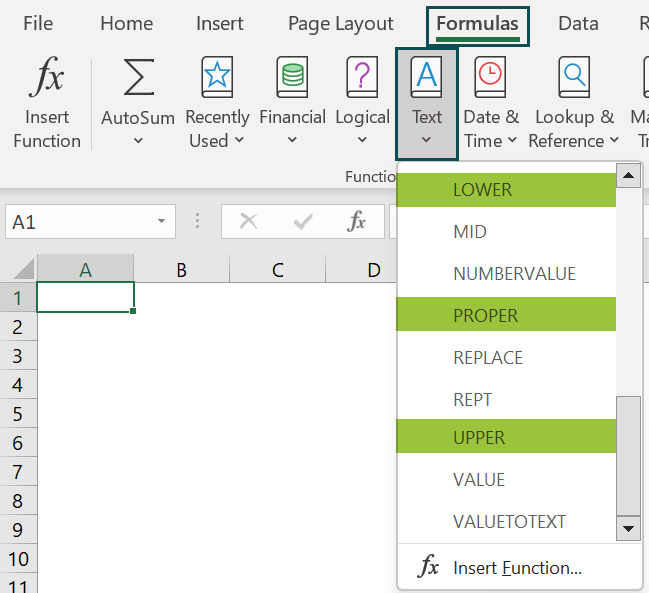2. How to set a required sentence case in Excel VBA?

We can set a required sentence case in Excel VBA in the following method. Let us see the steps with an example.

The table below contains text values.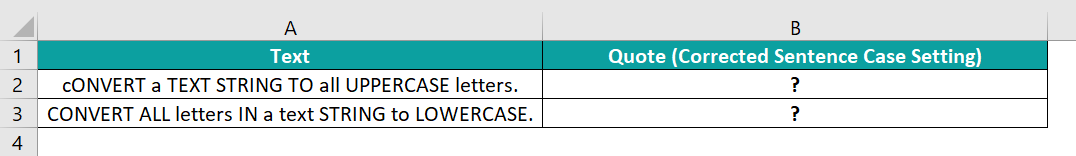Suppose the requirement is to show the given text values in cell range B2:B3, with the first letters in the two text values in uppercase and the remaining letters in lowercase.

Then, using Excel VBA here is how to achieve the required sentence case in the target cells.

• Step 1: With the active worksheet containing the above table open, open the VBA Editor using Alt + F11.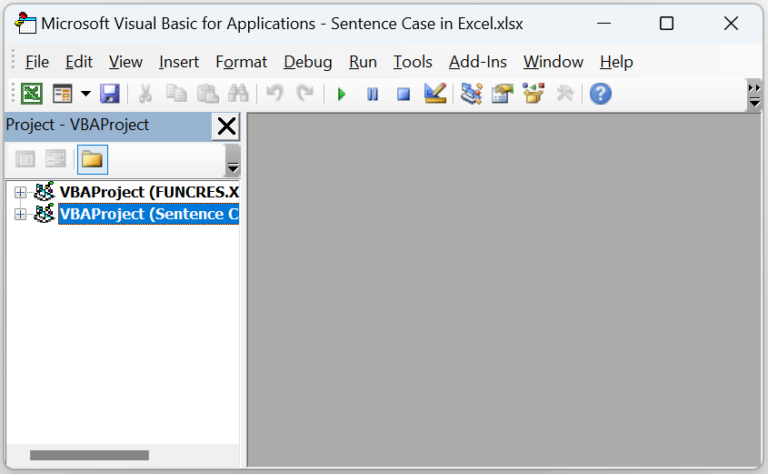• Step 2: Choose the required VBAProject and click the Module option in the Insert tab. It will open a new Module window.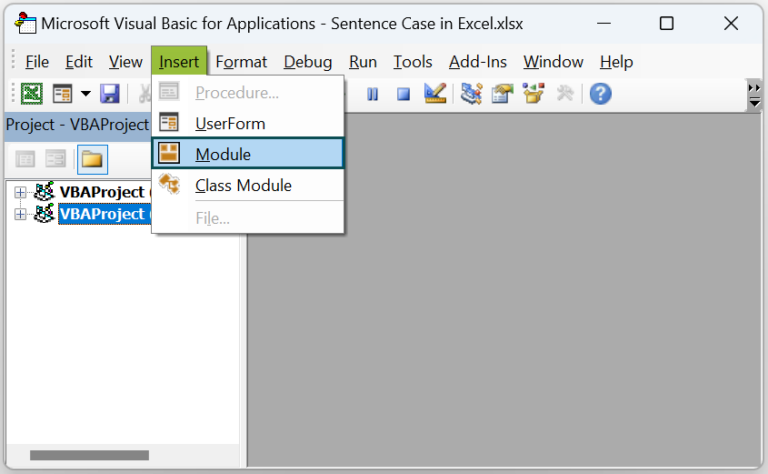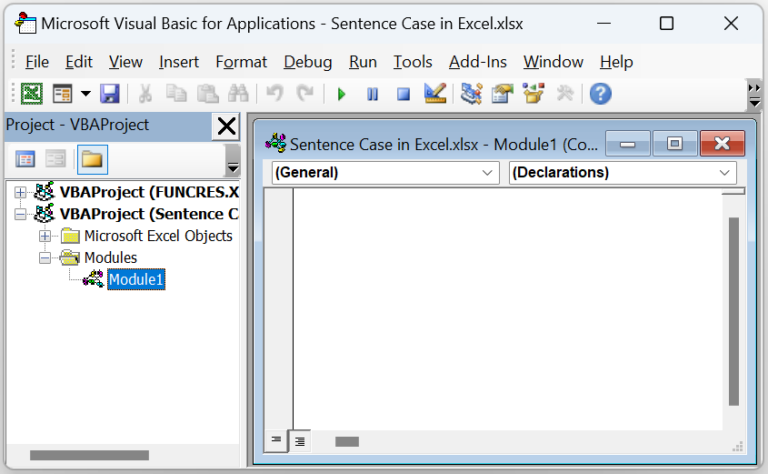• Step 3: Enter the VBA code in the Module window to update the required sentence case in the target cells.

Sub SentenceCase_fn()
Dim ws As Worksheet
Set ws = Worksheets(“Sentence_Case_FAQ”)
ws.Range(“B2”) = UCase(Left(ws.Range(“A2”), 1)) & LCase(Right(ws.Range(“A2”)) – 1)
ws.Range(“B3”) = UCase(Left(ws.Range(“A3”), 1)) & LCase(Right(ws.Range(“A3”)) – 1)
End Sub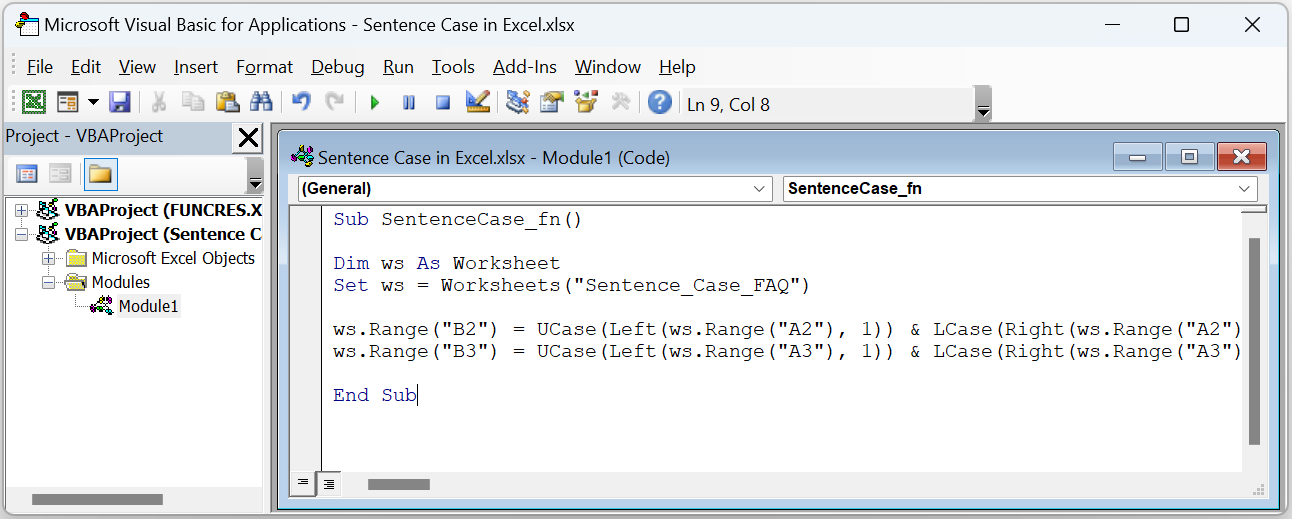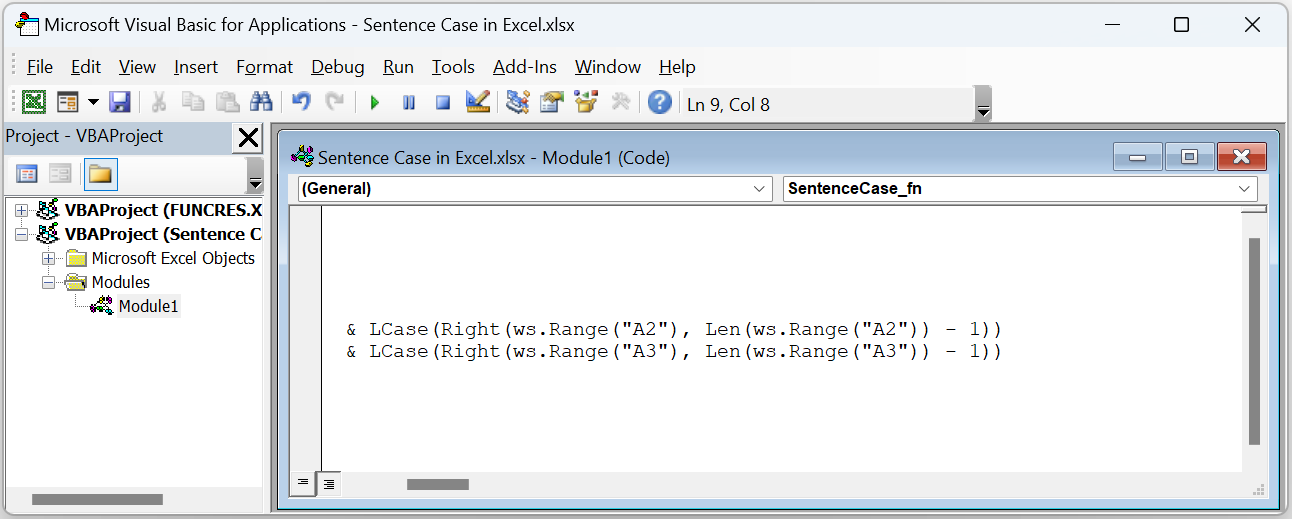• Step 4: Click the Run Sub/UserForm icon in the menu to run the code.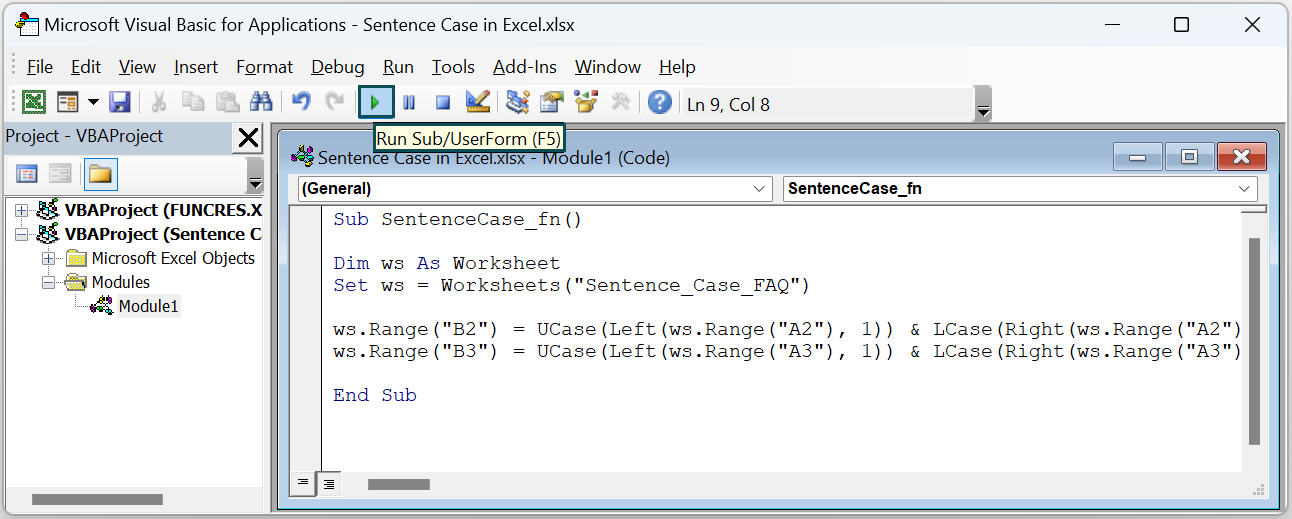Finally, open the active sheet to view the desired sentence case in the target cells.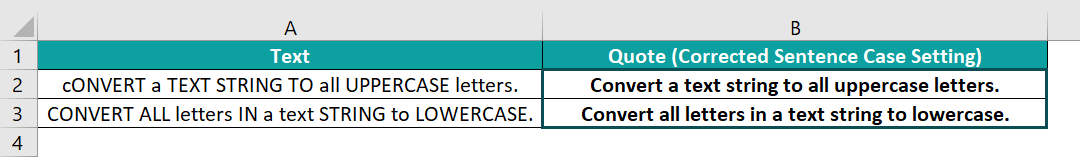The above VBA code uses a formula created using the methods for UPPER() [UCase()] and LOWER() [LCase()], with the LEFT(), RIGHT(), and LEN(). And the formula returns a text with its first character in uppercase and the remaining letters in lowercase.

3. What are the ways to apply Excel sentence cases without using formulas?

The ways to apply Excel sentence cases without using formulas are as follows:
The Flash Fill option in the Data tab.
Excel VBA codes to get shortcut icons in the Quick Access Toolbar and keyboard shortcuts.